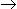# Aptitude - Numbers

Exercise : Numbers - General Questions
31.
On dividing a number by 5, we get 3 as remainder. What will the remainder when the square of the this number is divided by 5 ?
0
1
2
4
Explanation:

Let the number be x and on dividing x by 5, we get k as quotient and 3 as remainder.x = 5k + 3x2 = (5k + 3)2

= (25k2 + 30k + 9)

= 5(5k2 + 6k + 1) + 4On dividing x2 by 5, we get 4 as remainder.

32.
How many 3-digit numbers are completely divisible 6 ?
149
150
151
166
Explanation:

3-digit number divisible by 6 are: 102, 108, 114,... , 996

This is an A.P. in which a = 102, d = 6 and l = 996

Let the number of terms be n. Then tn = 996.a + (n - 1)d = 996102 + (n - 1) x 6 = 9966 x (n - 1) = 894(n - 1) = 149n = 150Number of terms = 150.

33.
How many natural numbers are there between 23 and 100 which are exactly divisible by 6 ?
8
11
12
13
None of these
Explanation:

Required numbers are 24, 30, 36, 42, ..., 96

This is an A.P. in which a = 24, d = 6 and l = 96

Let the number of terms in it be n.

Then tn = 96a + (n - 1)d = 9624 + (n - 1) x 6 = 96(n - 1) x 6 = 72(n - 1) = 12n = 13

Required number of numbers = 13.

34.
How many of the following numbers are divisible by 3 but not by 9 ?
2133, 2343, 3474, 4131, 5286, 5340, 6336, 7347, 8115, 9276
5
6
7
None of these
Explanation:

Marking (/) those which are are divisible by 3 by not by 9 and the others by (X), by taking the sum of digits, we get:s

21339 (X)

234312 (/)

347418 (X)

41319 (X)

528621 (/)

534012 (/)

633618 (X)

734721 (/)

811515 (/)

927624 (/)

Required number of numbers = 6.

35.
 (963 + 476)2 + (963 - 476)2 = ? (963 x 963 + 476 x 476)
1449
497
2
4
None of these